Function Repository Resource:

# HypergraphToGraph

Convert a hypergraph to a graph with the same distance matrix

Contributed by: Stephen Wolfram
 ResourceFunction["HypergraphToGraph"][hg] converts the ordered hypergraph hg to a directed graph with the same distance matrix.

## Details and Options

The hypergraph should be given as a list of lists, with one sublist for each hyperedge.
ResourceFunction["HypergraphToGraph"] takes the same options as Graph.

## Examples

### Basic Examples (2)

Convert a simple hypergraph to a graph:

 In:=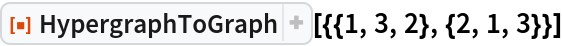Out=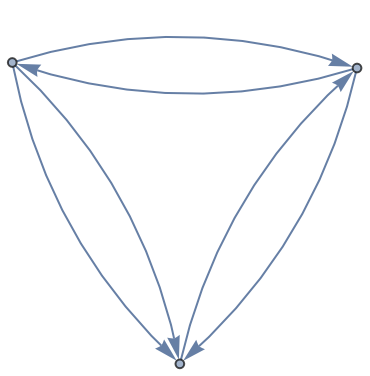Find the edge list of the resulting graph:

 In:=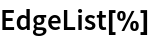Out=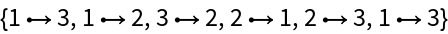Define a more complex hypergraph:

 In:=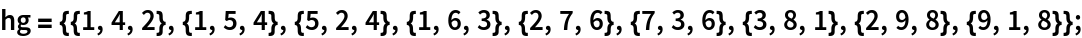Find the equivalent graph:

 In:=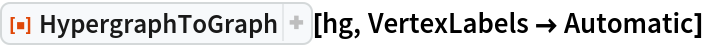Out=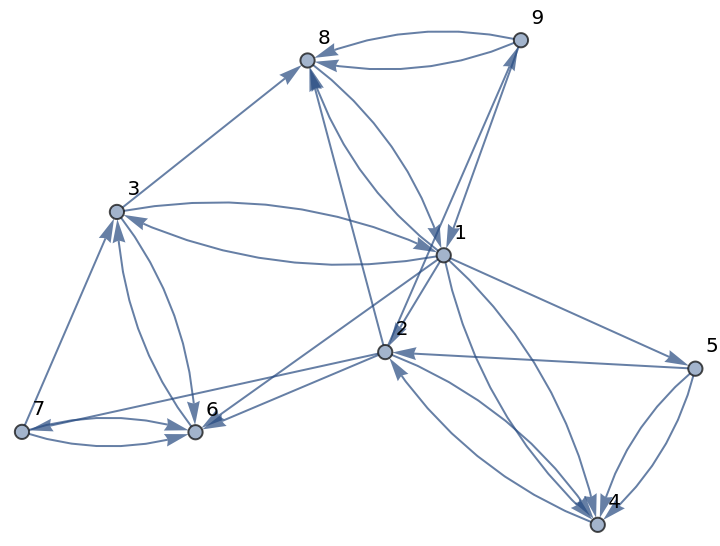## Version History

• 2.0.0 – 16 March 2020
• 1.0.0 – 04 December 2019